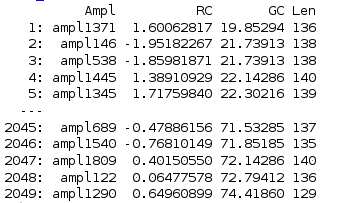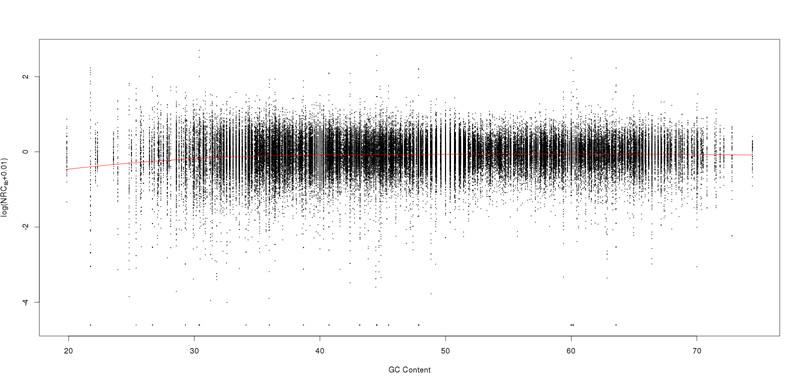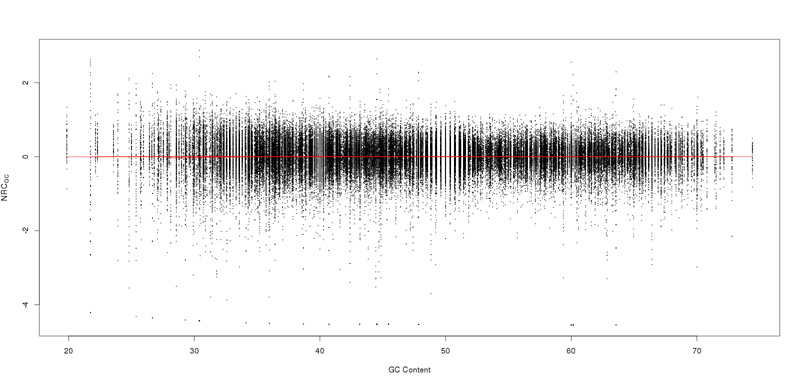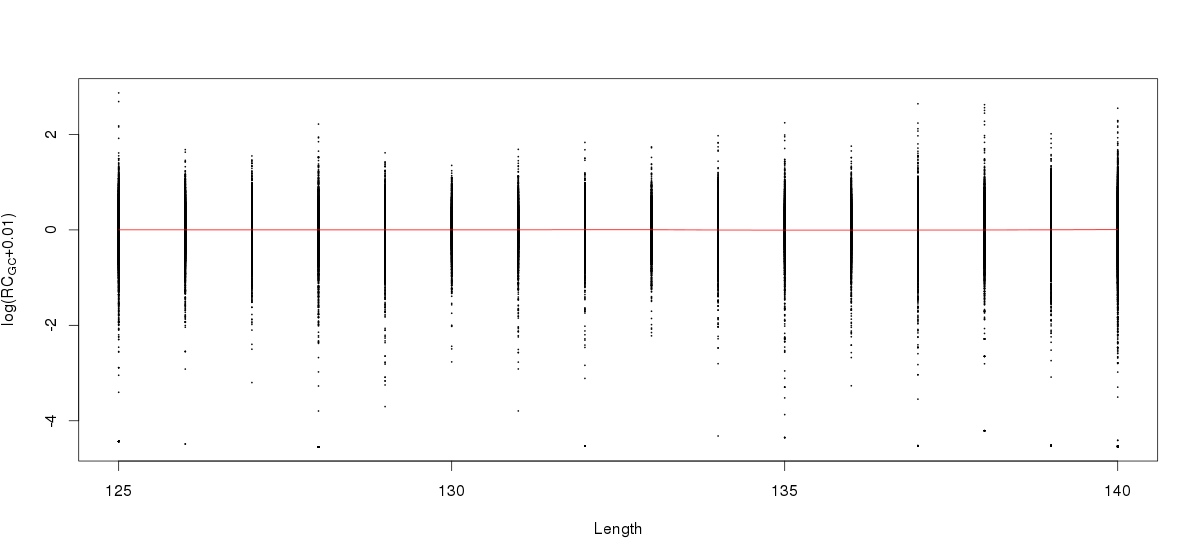# R语言利用loess如何去除某个变量对数据的影响详解

更新时间：2017年11月24日 08:45:01   作者：ywliao我要评论

R语言介绍

R语言是用于统计分析，图形表示和报告的编程语言和软件环境。 R语言由Ross Ihaka和Robert Gentleman在新西兰奥克兰大学创建，目前由R语言开发核心团队开发。

R语言的核心是解释计算机语言，其允许分支和循环以及使用函数的模块化编程。 R语言允许与以C，C ++，.Net，Python或FORTRAN语言编写的过程集成以提高效率。

R语言在GNU通用公共许可证下免费提供，并为各种操作系统（如Linux，Windows和Mac）提供预编译的二进制版本。
R是一个在GNU风格的副本左侧的自由软件，GNU项目的官方部分叫做GNU S.

R的演变

R语言最初是由新西兰奥克兰奥克兰大学统计系的Ross Ihaka和Robert Gentleman写的。 R语言于1993年首次亮相。

R的特点

• R语言是一种开发良好，简单有效的编程语言，包括条件，循环，用户定义的递归函数以及输入和输出设施。
• R语言具有有效的数据处理和存储设施，
• R语言提供了一套用于数组，列表，向量和矩阵计算的运算符。
• R语言为数据分析提供了大型，一致和集成的工具集合。
• R语言提供直接在计算机上或在纸张上打印的图形设施用于数据分析和显示。

当我们想研究不同sample的某个变量A之间的差异时，往往会因为其它一些变量B对该变量的固有影响，而影响不同sample变量A的比较，这个时候需要对sample变量A进行标准化之后才能进行比较。标准化的方法是对sample 的 A变量和B变量进行loess回归，拟合变量A关于变量B的函数 f(b)，f(b)则表示在B的影响下A的理论取值，A-f(B)（A对f(b）残差）就可以去掉B变量对A变量的影响,此时残差值就可以作为标准化的A值在不同sample之间进行比较。

Loess局部加权多项式回归

LOWESS最初由Cleveland 提出,后又被Cleveland&Devlin及其他许多人发展。在R中loess 函数是以lowess函数为基础的更复杂功能更强大的函数。主要思想为:在数据集合的每一点用低维多项式拟合数据点的一个子集,并估计该点附近自变量数据点所对应的因变量值,该多项式是用加权最小二乘法来拟合;离该点越远,权重越小,该点的回归函数值就是这个局部多项式来得到,而用于加权最小二乘回归的数据子集是由最近邻方法确定。

最大优点:不需要事先设定一个函数来对所有数据拟合一个模型。并且可以对同一数据进行多次不同的拟合，先对某个变量进行拟合，再对另一变量进行拟合，以探索数据中可能存在的某种关系，这是普通的回归拟合无法做到的。

LOESS平滑方法

1. 以x0为中心确定一个区间，区间的宽度可以灵活掌握。具体来说，区间的宽度取决于q=fn。其中q是参与局部回归观察值的个数，f是参加局部回归观察值的个数占观察值个数的比例,n是观察值的个数。在实际应用中，往往先选定f值，再根据f和n确定q的取值，一般情况下f的取值在1/3到2/3之间。q与f的取值一般没有确定的准则。增大q值或f值，会导致平滑值平滑程度增加，对于数据中前在的细微变化模式则分辨率低，但噪声小，而对数据中大的变化模式的表现则比较好；小的q值或f值，曲线粗糙，分辨率高，但噪声大。没有一个标准的f值，比较明智的做法是不断的调试比较。

2. 定义区间内所有点的权数，权数由权数函数来确定，比如立方加权函数weight = (1 - (dist/maxdist)^3)^3),dist为距离x的距离，maxdist为区间内距离x的最大距离。任一点(x0,y0)的权数是权数函数曲线的高度。权数函数应包括以下三个方面特性：(1)加权函数上的点(x0,y0)具有最大权数。(2)当x离开x0(时，权数逐渐减少。(3)加权函数以x0为中心对称。

3. 对区间内的散点拟合一条曲线y=f(x)。拟合的直线反映直线关系，接近x0的点在直线的拟合中起到主要的作用，区间外的点它们的权数为零。

4. x0的平滑点就是x0在拟合出来的直线上的拟合点(y0,f( x0))。

5. 对所有的点求出平滑点，将平滑点连接就得到Loess回归曲线。

R语言代码

``` loess(formula, data, weights, subset, na.action, model = FALSE,
span = 0.75, enp.target, degree = 2,
parametric = FALSE, drop.square = FALSE, normalize = TRUE,
family = c("gaussian", "symmetric"),
method = c("loess", "model.frame"),
control = loess.control(...), ...)```

formula是公式，比如y~x,可以输入1到4个变量;

data是放着变量的数据框，如果data为空，则在环境中寻找;

na.action指定对NA数据的处理，默认是getOption("na.action");

model是否返回模型框；

span是alpha参数，可以控制平滑度,相当于上面所述的f，对于alpha小于1的时候，区间包含alpha的点，加权函数为立方加权，大于1时，使用所有的点，最大距离为alpha^(1/p)，p 为解释变量;

anp.target，定义span的备选方法；

normalize，对多变量normalize到同一scale；

family，如果是gaussian则使用最小二乘法，如果是symmetric则使用双权函数进行再下降的M估计；

method，是适应模型或者仅仅提取模型框架；

control进一步更高级的控制，使用loess.control的参数；

其它参数请自己参见manual并且查找资料

```loess.control(surface = c("interpolate", "direct"),
statistics = c("approximate", "exact"),
trace.hat = c("exact", "approximate"),
cell = 0.2, iterations = 4, ...)```

surface，拟合表面是从kd数进行插值还是进行精确计算；

statistics,统计数据是精确计算还是近似，精确计算很慢

trace.hat,要跟踪的平滑的矩阵精确计算或近似？建议使用超过1000个数据点逼近，

cell,如果通过kd树最大的点进行插值的近似。大于cell floor(nspancell)的点被细分。

robust fitting使用的迭代次数。

```predict(object, newdata = NULL, se = FALSE,
na.action = na.pass, ...)```

object，使用loess拟合出来的对象；

newdata,可选数据框，在里面寻找变量并进行预测；

se,是否计算标准误差；

对NA值的处理

生物数据分析中，我们想查看PCR扩增出来的扩增子的测序深度之间的差异，但不同的扩增子的扩增效率受到GC含量的影响，因此我们首先应该排除掉GC含量对扩增子深度的影响。

amplicon 测序数据，处理后得到的每个amplicon的深度，每个amplicon的GC含量，每个amplicon的长度`gcCount.loess <- loess(log(RC+0.01)~GC,data=RC_DT,control = loess.control(surface = "direct"),degree=2)`

```predictions1<- predict (gcCount.loess,RC_DT\$GC)
#plot scatter and line
plot(RC_DT\$GC,log(RC_DT\$RC+0.01),cex=0.1,xlab="GC Content",ylab=expression(paste("log(NRC"["lib"],"+0.01)",sep="")))
lines(RC_DT\$GC,predictions1,col = "red")``````#sustract the influence of GC
resi <- log(RC_DT\$RC+0.01)-predictions1
RC_DT\$RC <- resi
setkey(RC_DT,GC)```

```#plot scatter and line using Norm GC data
plot(RC_DT\$GC,RC_DT\$RC,cex=0.1,xlab="GC Content",ylab=expression("NRC"["GC"]))
gcCount.loess <- loess(RC~GC,data=RC_DT,control = loess.control(surface = "direct"),degree=2)
save(gcCount.loess,file="/home/ywliao/project/Gengyan/gcCount.loess.Robject")
predictions2 <- predict(gcCount.loess,RC_DT\$GC)
lines(RC_DT\$GC,predictions2,col="red")
save(RC_DT,file="/home/ywliao/project/Gengyan/RC_DT.Rdata")``````library(data.table)

RRC_DT <- RC_DT[Type=="WBC" & !is.na(RC),]

lst <- list()
for (Samp in unique(RC_DT\$Sample)){
RC_DT <- RRC_DT[Sample==Samp]
####loess GC vs RC####
gcCount.loess <- loess(log(RC+0.01)~GC,data=RC_DT,control = loess.control(surface = "direct"),degree=2)
predictions1<- predict (gcCount.loess,RC_DT\$GC)
#plot scatter and line
#plot(RC_DT\$GC,log(RC_DT\$RC+0.01),cex=0.1,xlab="GC Content",ylab=expression(paste("log(NRC"["lib"],"+0.01)",sep="")))
#lines(RC_DT\$GC,predictions1,col = "red")
#sustract the influence of GC
resi <- log(RC_DT\$RC+0.01)-predictions1
RC_DT\$NRC <- resi
setkey(RC_DT,GC)
#plot scatter and line using Norm GC data
#plot(RC_DT\$GC,RC_DT\$NRC,cex=0.1,xlab="GC Content",ylab=expression("NRC"["GC"]))
gcCount.loess <- loess(NRC~GC,data=RC_DT,control = loess.control(surface = "direct"),degree=2)
predictions2 <- predict(gcCount.loess,RC_DT\$GC)
#lines(RC_DT\$GC,predictions2,col="red")
lst[[Samp]] <- RC_DT
}
NRC_DT <- rbindlist(lst)
save(RC_DT,file="/home/ywliao/project/Gengyan/NRC_DT.Rdata")

####loess len vs RC###
setkey(RC_DT,Len)
len.loess <- loess(RC_DT\$NRC~RC_DT\$Len, control = loess.control(surface = "direct"),degree=2)
predictions2<- predict (len.loess,RC_DT\$Len)
#plot scatter and line
plot(RC_DT\$Len,RC_DT\$NRC,cex=0.1,xlab="Length",ylab=expression(paste("log(RC"["GC"],"+0.01)",sep="")))
lines(RC_DT\$Len,predictions2,col = "red")```